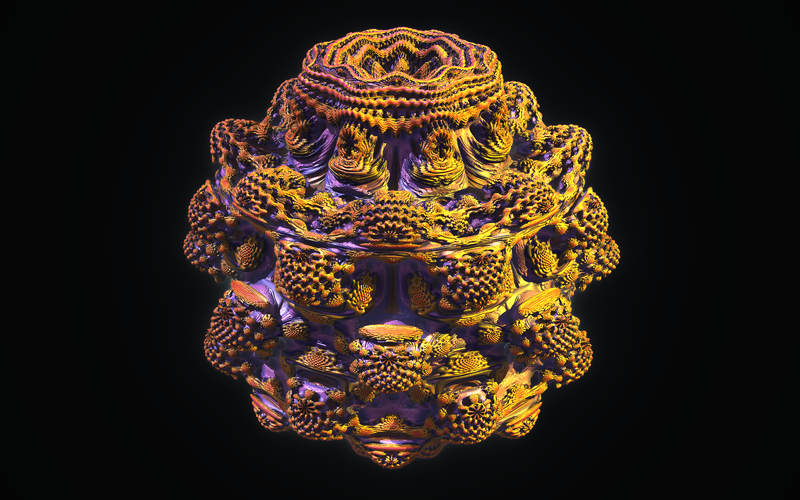## The Mandelbulb

Here is a 3200x2000 pixel background picture of the mandelbulb set. It was rendered with the free program "mandelbulber" under linux (apt-get install mandelbulber). The Mandelbulb is the set of points C=(x,y,z) in space for which the iteration of the map T(X)=X2 + C starting at 0=(0,0,0) does not lead to infinity. The square X2 of a point is defined as the point with spherical coordinates (r2,2 t,2s) if (r,t,s) are the spherical coordinates of X. Despite the fact that the set is extremely beautiful, almost nothing is known about it. Is it connected, simply connected?